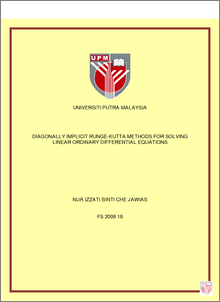# Diagonally Implicit Runge-Kutta Methods For Solving Linear Ordinary Differential Equations

## Citation

Che Jawias, Nur Izzati (2009) Diagonally Implicit Runge-Kutta Methods For Solving Linear Ordinary Differential Equations. Masters thesis, Universiti Putra Malaysia.

## Abstract

This thesis deals with the derivation of diagonally implicit Runge-Kutta (DIRK) methods which are specially designed for the integration of linear ordinary differential equations (LODEs). The restriction to LODEs with constant coefficients reduces the number of order equations which the coefficients of Runge-Kutta (RK) methods must satisfy. This freedom is used to construct new methods which are more efficient compared to the conventional RK methods. Having achieved a particular order of accuracy, the best strategy for practical purposes would be to choose the coefficients of the RK methods such that the error norm is minimized. The free parameters chosen are obtained from the minimized error norm. This resulted in methods which are almost one order higher than the actual order. In this thesis we construct a fourth order DIRK method without taking into account the error norm. We also construct fourth and fifth order DIRK methods using the minimized error norm. The stability aspects of the methods are investigated by finding the stability polynomials of the methods, which are then solved to obtain the stability regions using MATHEMATICA package. The methods are found to have bigger regions of stability compared to the explicit Runge-Kutta (ERK) methods of the same type (designed for the integration of LODEs). Later, we built codes using C++ programming based on the methods. Sets of test problems on linear ordinary differential equations are used to validate the methods and numerical results show that the new methods produce smaller global error compared to ERK methods. From the stability regions and numerical results obtained, we can conclude that the new DIRK methods are more stable and more accurate compared to the explicit one. Higher order methods also gives better result compared to lower order methods.Preview
PDF
A__FS_2009_18.pdfView Item